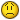Making MAX and MIN range variable

D

Dano

I have a project where I have columns of data where I will need to
find the MAX and MIN functions. The data is in order (newest first).
I often need to just find the corresponding MAX and MIN for different
ranges within each column (i.e. 20 data points, 50 , or 100 ).However
the starting poing of each range in my MAX and MIN is constant

.. I would like to be able to put the length the range as a variable
in a cell and have the MAX and MIN function reference that variable
when determining the range of each function.

Foe example, if the data is in Column B the function would look like
this =MAX(\$B\$1B1+ Number in variable reference))

Thanks so much

Dano

Y

ytayta555

Foe example, if the data is in Column B the function would look like
this =MAX(\$B\$1B1+ Number in variable reference))

You can do this with a macro , but I need see your data in
Worksheet . If desire , send me to my g-mail , or to
(e-mail address removed) the Workbook in xls. format ,
I use 2003 . Then , I'll can post the macro here .